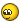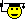# Feed phase center

In order to tune the receiving system to the global maximum sensitivity, you first need to match the angle of exposure of the antenna with its angular opening (sometimes they say "angular aperture", although this is not correct, IMHO), and then precisely align the antenna to the satellite.

If the LNB is not in the geometric focus of the parabolic antenna, then we will adjust the antenna not by the true azimuth and elevation of the satellite, but by choosing the "best" position of the LNB around this initially incorrect point, we will tune to the maximum multi-feed pseudofocus.

Do it right like this:

- according to the calculated elevation angle of the vertex satellite and the offset angle of the mirror, set the deviation from the vertical of the parabolic mirror cut and do not touch this adjustment in the future;

- install LNB strictly in the plane of symmetry of the parabolic mirror and direct the axis of the first one to the aiming point on the second one;

- by changing the antenna azimuth within +/- 3° from the calculated one to the summit satellite, adjust this parameter to the maximum signal and do not touch this adjustment in the future;

- moving the LNB along the line of sight to achieve the maximum signal. At this moment, the geometric focus of the parabolic mirror will be aligned with the phase center of the feed.

According to my experiments with LNB Titanium TSX, Inverto Black Eco, Inverto Black Ultra, Megasat, Orton, etc., their phase center is in the plane of the cut of the plastic casing where it passes to a diameter of 40mm. This is approximately in the neck of the conical horn or in the middle of the mod transformer as in the Inverto Black Ultra.

Some theory of wave propagation:

Quote

The eikonal equation (ancient Greek εἰκών) is a non-linear partial differential equation encountered in wave propagation problems when the wave equation is approximated using WKB theory. It is a consequence of Maxwell's equations, and connects wave optics with geometric optics

Recall that light is electromagnetic waves with a very short wavelength, but this wave process is much closer to the Ku range than the waves that propagated in a cantilevered beam and, thanks to Poisson's brilliant solution, laid the foundation for the theory of wave propagation.

Quote

Geometric optics is the branch of optics that considers the wavelength to be negligible. The basis of geometric optics is the eikonal equation.

It follows from the eikonal equation that geometric optics is applicable only for short wavelengths. The shorter the wavelength, the more accurate the geometrical optics approximation.

It follows from the solution of the eikonal equation that the wave process propagates along the ray tubes:where r(s) is the beam, is the cross section of the beam tube.

Of the properties of a ray tube, the most important for us is that the wave energy propagates exclusively along the ray tube, without flowing through its side walls, and the energy density inside the ray tube is inversely proportional to its cross-sectional area .

A ray tube can be compared to a river in which the water does not flow over the banks and which flows slowly and smoothly where the river is wide and deep, but rushes with a roar in the gorge, where the banks converge between the cliffs ...

Let's ask ourselves the question: "What is the minimum radius of the ray tube?" - by answering which, we will get the key to solving the problem about the position of the phase center (PC) of the irradiator

I have derived a formula for determining the position of the phase center relative to the horn:

∆Z=0.293λ/sin(α/2),

where

∆Z - displacement of the PC from the top of the cone inscribed in the receiving horn;

λ - wavelength;

α - opening angle of the cone.

Based on the results of practical tuning of the long-focus antenna CA-902 with LNB Inverto Black Ultra, I was convinced that the best alignment results are obtained when the geometric focus of the mirror is on the feed axis in the cut plane of the back wall of the LNB feed housing, where the diameter begins 40mm.

In the drawing, this plane is marked with a blue dotted line (at a distance of 26 mm from the irradiator cut):The figure shows the results of calculating the DP of a corrugated irradiator using the SABOR program, a frontal photo of the Inverto Black Ultra irradiator and a drawing of its longitudinal section according to the measurements I made using a caliper.

In the drawing, the irradiator aperture cone Alpha=62° is limited by gray lines -10dB Width 69.4° at 12.44GHz

The position of the phase center (ФЦ) of the irradiator is shown by red circles for the extreme frequencies of the range 10.7 and 12.75 GHz (Lambda = 28 and 23.5 mm, respectively)

The distance from the phase center to the top of the cone dXфц is calculated according to my formula, where Lambda is the wavelength of the corresponding frequency in free space (not in the waveguide!)

As you can see, the best LNB alignment results were achieved with alignment of the phase center for the highest frequency of the range with the geometric focus of the parabolic mirror,

moreover, the axis of the irradiator lies on the line of sight

Let's summarize:

1. A wave propagates along a beam only when its frequency is infinite.

This is confirmed in geometric optics, where the frequency of an electromagnetic wave tends to infinity.

2. A wave with a finite frequency (as in our case) propagates along the ray tube, and the energy of the electromagnetic wave cannot flow through the "walls" of the ray tube.

3. There is a minimum diameter of a ray tube in which an electromagnetic wave with a given wavelength can propagate. This takes into account the coefficient in front of the Lambda in the formula I derived based on the solution of the eikonal equation.

4. The phase center is defined as the point of intersection of the axes of the extreme wave tubes in the feed. The red dots show the phase centers for a wavelength of 28mm (ФЦ-28) and 23.5mm (ФЦ-23.5) - this is for the cutoff frequencies of the Ku band.

5. The opening angle of the irradiator is 62°.

6. The correctness of my formula for determining the position of the phase center is confirmed by the practical results of adjusting my antennas.

7. The accuracy of the formula is confirmed by the results of computer simulation of wave fields.

The calculations in the Ansys HFSS program according to my data were performed by Yury Vitalievich Pilipenko, for which I respect him and thank you very much.In the figure, thin black circles show the lines of equal phases of the wave field (isophase surfaces).

Their centers coincide and are in the phase center of the irradiator (yellow circle)

As you can see with the naked eye, the position of the phase center in the figure perfectly matches the one calculated by my formulaThe animation shows the process of emitting a wave field from the irradiator with the geometry of Inverto Black Ultra:Unfortunately, in the Ansys HFSS program, the center of NOT isophase, but isoamplitude lines is determined, which is erroneously called the phase center of the feedThis can be clearly seen in the animation, which shows two spheres centered at the "pseudo phase center" (white circle or red cross), which does NOT coincide with the true PC (ФЦ) :